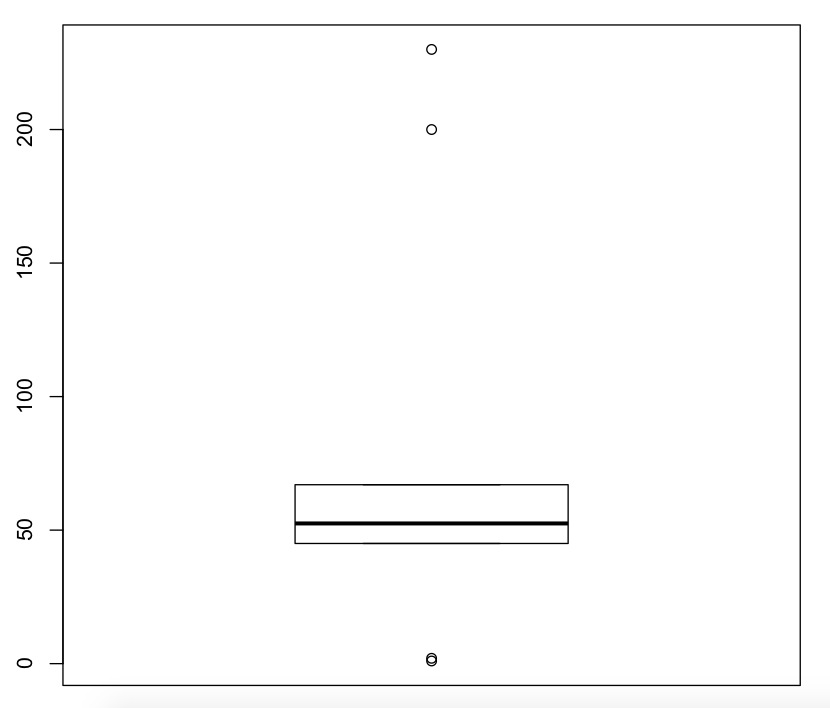What is an Outlier?

Extreme low values and extremely high values will be called as outliers.
These values affect the decision. These values also called as noise in a dataset.

From Wikipedia.

In statistics, an outlier is an observation point that is distant from other observations. An outlier may be due to variability in the measurement or it may indicate an experimental error; the latter are sometimes excluded from the data set. An outlier can cause serious problems in statistical analyses

Say, for example, take a total sales of the last ten days of some x company.

1,2,50,45,67,200,230,55,56,49

Total sales in thousands.

From the above dataset, you can find that some days very poor sale and some days too high sales. These are called as an outlier. But actually, the sale value is around 50 in most of the times. But if you find out the mean of above value it is 75.5. So this is a false assumption due to the noise present in the data. If you are not treating these outliers, then you will end up producing the wrong results. That's why it is very important to process the outlier.

You can see whether your data had an outlier or not using the boxplot in r programming.

``````sales=c(1,2,50,45,67,200,230,55,56,49)
boxplot(sales)``````Sale Boxplot Diagram.

From the diagram, if you see any dot above and below, then your data had an outlier.

To find out outlier values.

``````#find outliers values
OutVals = boxplot(sales)\$out

#print outlier
OutVals
   1   2 200 230``````

Get the index position of outlier using the which function.

``````#find outlier index position in vector
which(sales %in% OutVals)

 1 2 6 7``````

Remove outlier from your dataset use the below code.

``sales[ !(sales %in%OutVals) ]``